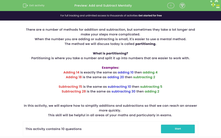### Comprehensive & curriculum aligned

In this worksheet, students will practise partitioning into tens and units, adding tens and counting forwards or back for quick mental computations in exams.Key stage:  KS 4

Year:  GCSE

GCSE Subjects:   Maths

GCSE Boards:   Pearson Edexcel, OCR, Eduqas, AQA,

Curriculum topic:   Number, Number Operations and Integers

Curriculum subtopic:   Structure and Calculation Calculations with Integers

Difficulty level:#### Worksheet Overview

There are a number of methods for addition and subtraction, but sometimes they take a lot longer and make your steps more complicated.

When the number you are adding or subtracting is small, it's easier to use a mental method.

The method we will discuss today is called partitioning.

What is partitioning?

Partitioning is where you take a number and split it up into numbers that are easier to work with.

Examples:

Subtracting 15 is the same as subtracting 10 then subtracting 5

Subtracting 28 is the same as subtracting 30 then adding 2

In this activity, we will explore how to simplify additions and subtractions so that we can reach an answer more quickly.

This skill will be helpful in all areas of your maths and particularly in exams.

### What is EdPlace?

We're your National Curriculum aligned online education content provider helping each child succeed in English, maths and science from year 1 to GCSE. With an EdPlace account you’ll be able to track and measure progress, helping each child achieve their best. We build confidence and attainment by personalising each child’s learning at a level that suits them.

Get started